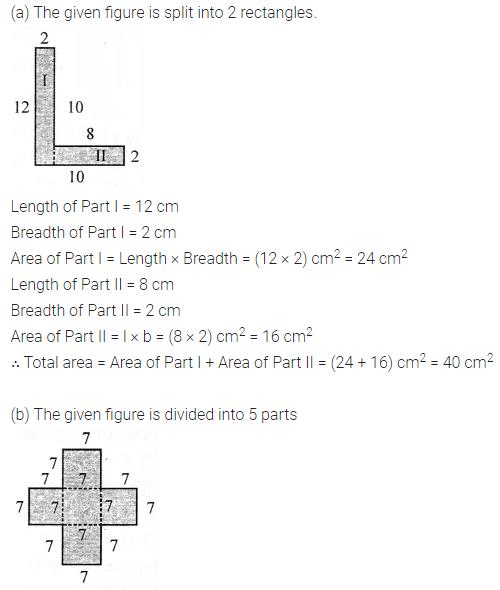ML Aggarwal Class 6 Solutions Chapter 14 Mensuration Ex 14.2 for ICSE Understanding Mathematics acts as the best resource during your learning and helps you score well in your exams.

## ML Aggarwal Class 6 Solutions for ICSE Maths Chapter 14 Mensuration Ex 14.2

Question 1.
Find the area of the region enclosed by the following figures by counting squares: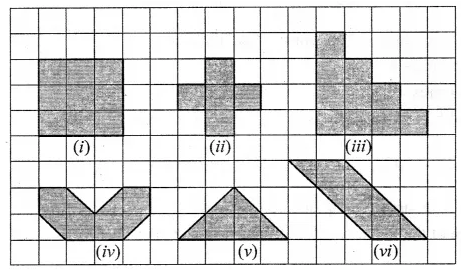Solution: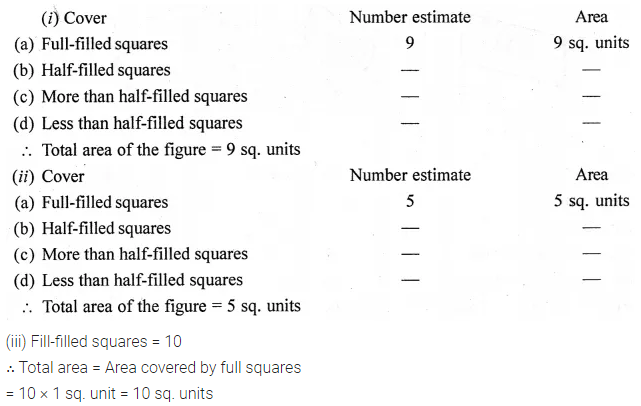Question 2.
Find the area of the following closed figures by counting squares:Solution: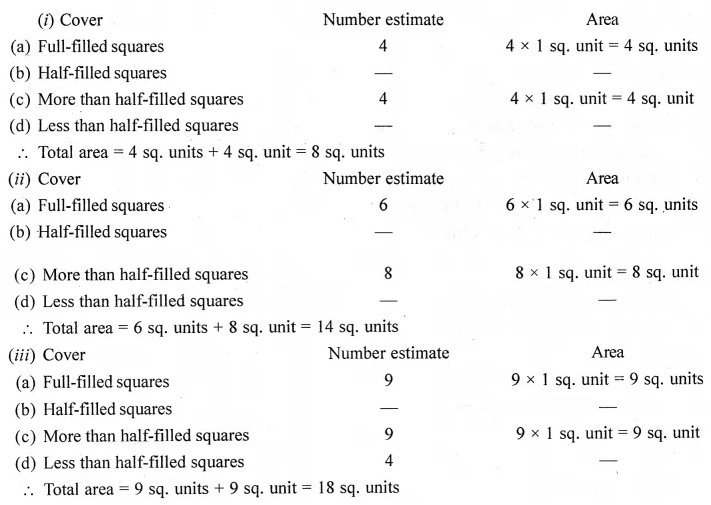Question 3.
Find the areas of the rectangles whose lengths and breadths are:
(i) 9 m and 6 m
(ii) 17 m and 3 m
(iii) 14 m and 4 m
Which one has the largest area and which one has the smallest area?
Solution: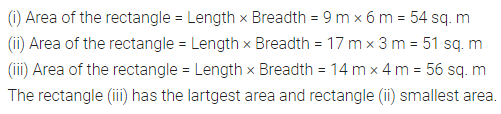Question 4.
Find the areas of the rectangles whose two adjacent sides are:
(i) 14 cm and 23 cm
(ii) 3 km and 4 km
(iii) 2 m and 90 cm
Solution: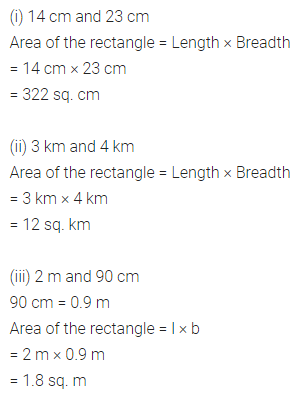Question 5.
Find the areas of the squares whose sides are:
(i) 8 cm
(ii) 14 m
(iii) 2 m 50 cm
Solution:Question 6.
A room is 4 m long and 3 m 25 cm wide. How many square metres of carpet is needed to cover the floor of the room?
Solution: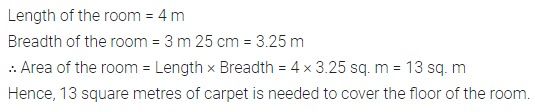Question 7.
What is the cost of tiling a rectangular field 500 m long and 200 m wide at the rate of ₹7.5 per hundred square metres?
Solution:Question 8.
A floor is 5 m long and 4 m wide. A square carpet of sides 3 m is laid on the floor. Find the area of the floor that is not carpeted.
Solution: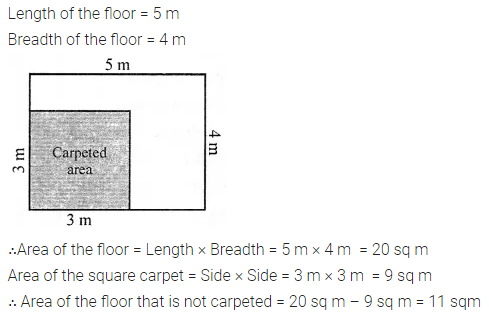Question 9.
In the given figure, find the area of the path (shown shaded) which is 2 m wide all around.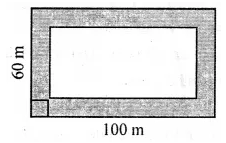Solution: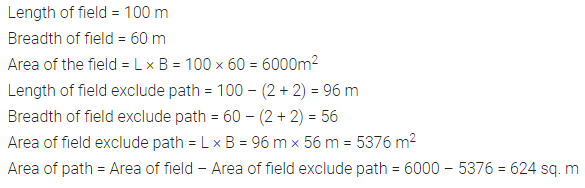Question 10.
Four square flower beds of side 1 m 50 cm are dug on a rectangular piece of land 8 m long and 6 m 50 cm wide. What is the area of the remaining part of the land?
Solution: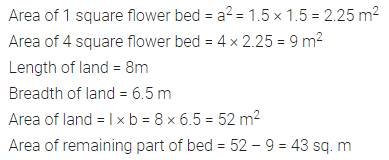Question 11.
How many tiles whose length and breadth are 12 cm and 5 cm respectively will be needed to cover a rectangular region whose length and breadth are respectively :
(i) 70 cm and 36 cm
(ii) 144 cm and 1 m.
Solution: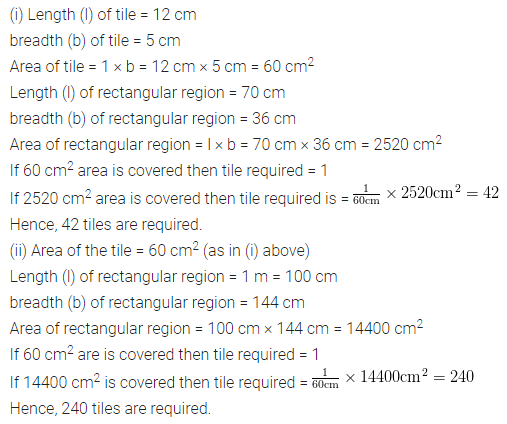Question 12.
The area of a rectangular plot is 340 sq. m. If its breadth is 17 m, find its length and the perimeter.
Solution: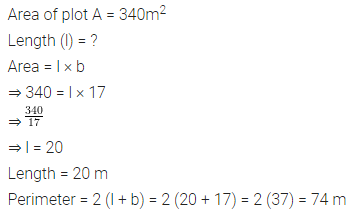Question 13.
If the area of a rectangular plot is 144 sq. m and its length is 16 m. Find the breadth of the plot and the cost of fencing it at the rate of ₹ 6 per metre.
Solution: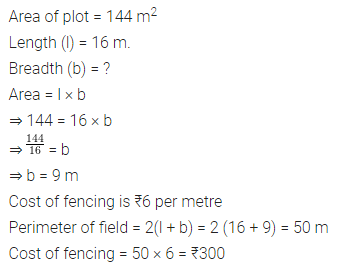Question 14.
Split the following shapes into rectangles and find their areas. (The measures are given in centimetres).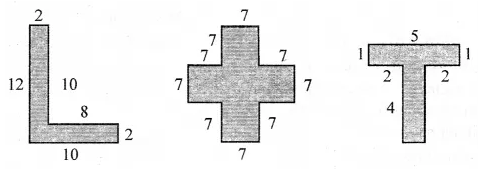Solution: